Once you have run an experiment on EcoCommons, the results page shows you a variety of different outputs. Below is an explanation on how to interpret the outputs of a Species Distribution Model experiment.

## Predicted distribution map

The primary output of a Species Distribution Model is the map that shows the predicted distribution of your species under the baseline conditions. It is important to note that this not really a prediction of where the species occurs, but rather the distribution of suitable habitat as defined by the environmental variables that you included in the model. These baseline conditions can include current climate conditions, but also other environmental variables such as soil pH or vegetation type. The prediction is visualised as the suitability of a grid cell on a scale from 0 to 1, where 0 refers to very low suitability and 1 refers to very high suitability.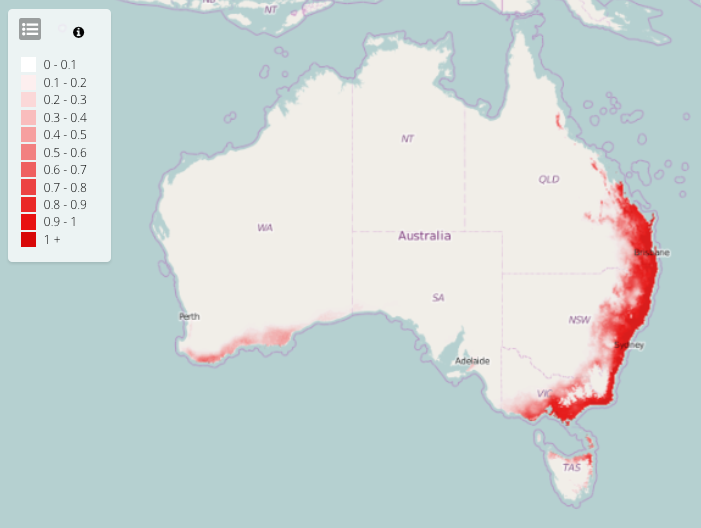## Constrained and Unconstrained projection maps

There are two predicted distribution maps produced from a SDM experiment except when categorical or freshwater variables are used. The first is the constrained projection map which only shows the predicted habitat suitability within the constraint selected for the experiment. The second map shows the unconstrained projection map which shows the predicted habitat suitability across the entire area with climate/environment variables.## Pseudo-absence points map

This map shows the pseudo-absence (or background) points generated to calibrate the model in this case no true absence data were available. The pseudo-absence points are generated in the smallest geographical area defined either by the geographical extent of the environmental layers, or by the extent defined in the ‘Constraints’ tab of the SDM experiment.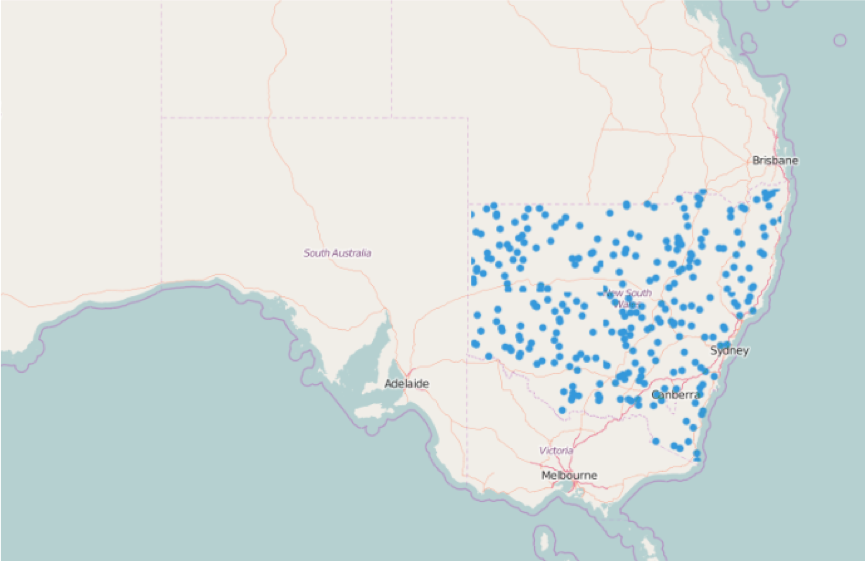## Model evaluation statistics

The table of model evaluation statistics shows various evaluation criteria that can be used to measure the performance of the model. A more detailed support article on model evaluation will follow soon.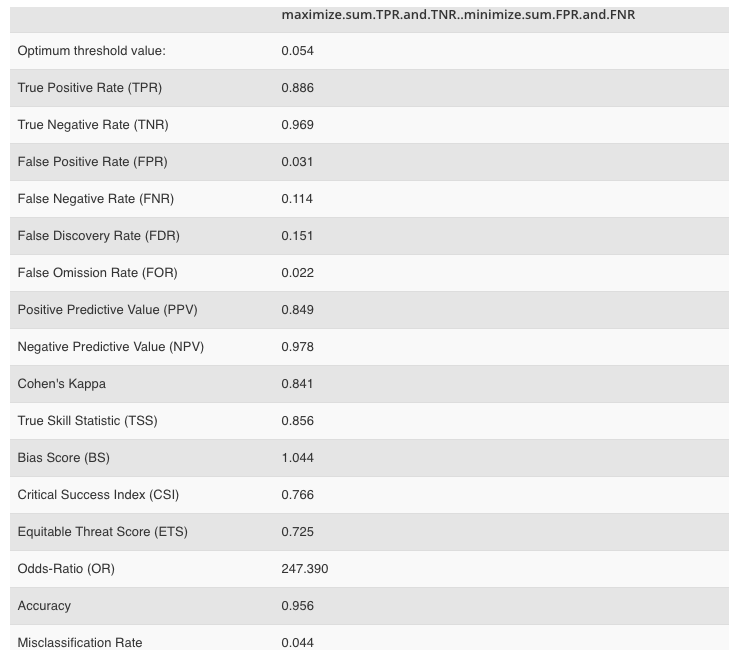## Response curves

These plots show the relationship between the probability of occurrence for a species and each of the environmental variables. For each plot, the response is modelled for one environmental variable while the other environmental variables are held constant at their mean. The x-axis represents the range of values of the environmental variable, and the y-axis gives the probability of occurrence on a scale from 0 (low probability) to 1 (high probability). The response curves in this plot show that the probability of occurrence of this species follows an optimum curve for the annual mean temperature (bioclim_01) with a high probability in areas that have a temperature between ~17 and 22 degrees Celsius. For the variable annual precipitation (bioclim_12), the curve shows a steep decline with increasing precipitation, indicating that this species likely prefers drier areas.## Presence/absence density plot

This plot shows the probability density of presences and absences across the range of threshold probability values. For each threshold probability value, the curve (blue for presences; red for absences) indicates the proportion of all observed presence/absence points that are predicted to be present/absent by the model.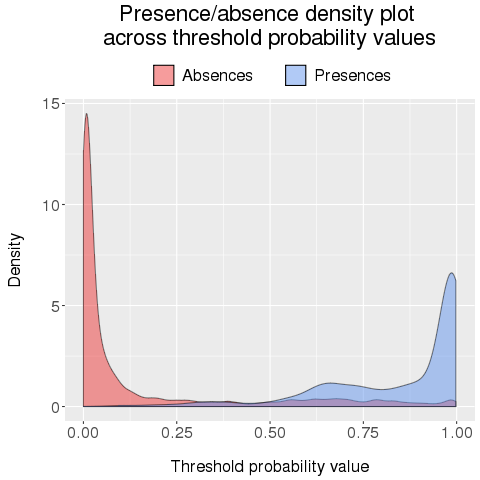## Presence/absence histogram

This plot shows a histogram with the number of predicted presences (blue) and absences (red) across the range of threshold probability values.## Sensitivity/Specificity plot

This plot shows the values of the True Positive Rate (Sensitivity) and the True Negative Rate (Specificity) across the range of the threshold probability values. See Model evaluation (coming soon) for a description of TPR (True Positive Rate) and TNR (True Negative Rate ).## Error rates plot

This plot shows the values of four different error rates across the range of threshold probability values.

The False Positive Rate (FPR) and False Negative Rate (FNR) are diagnostic errors, which represent the proportion of observed absences and presences that are predicted incorrectly by the model. These error rates give us information about how often the model is wrong relative to our pre-existing knowledge about the observed value of a location.

The False Discovery Rate (FDR) and False Omission Rate (FOR) are predictive errors, which represent the proportion of predicted presences and absences that are incorrect. These error rates inform us how likely it is that the prediction for a particular location is actually true, for example, if the model predicts a rare species to be present in a location, how likely is that to be true?## Relative Operating Characteristics (ROC) plot

The ROC plot is a graph with the False Positive Rate (1-Specificity) on the x-axis and the True Positive Rate (Sensitivity) on the y-axis plotted across the range of threshold probability values. The closer the ROC curve follows the y-axis, the larger the area under the curve, and thus the more accurate the model. A random guess would result in a point along the grey diagonal line from the left bottom to the right corner.

The value for ROC is the area under the curve (AUC), and is calculated by summing the area under the ROC curve. A value of 0.5 represents a random prediction, and thus values above 0.5 indicate predictions better than random. In general, AUC values of 0.5–0.7 are considered low and represent poor model performance, values of 0.7–0.9 are considered moderate, and values above 0.9 represent excellent model performance.## Loss functions plot

This plot shows the values of 4 different loss functions across the range of threshold probability values. These loss functions represent different methods to balance the error rates. We distinguish two types of error rates:

1. Diagnostic (producer) errors (False Positive Rate and False Negative Rate): these represent the proportion of incorrect predictions given a certain observed value.

2. Predictive (user) errors (False Discovery Rate and False Omission Rate): these represent the chance that given a certain prediction by the model, the actual value will be different?

The different loss functions in the plot are:

1. Maximize (TPR + TNR) = minimizing the diagnostic errors (FPR and FNR).

2. Maximize (PPV + NPV) = minimizing the predictive errors (FDR and FOR).

3. Balance all errors (¼(FPR + FNR + FDR + FOR): consider all error rates in a balanced approach.

4. Equalize diagnostic errors (FPR = FNR) = equalize TPR and TNR.## Loss function intervals table

This table shows the lower, upper and best threshold probability value for the conversion of probability of occurrence measures to binary presence/absence outputs for 4 different loss functions: 1) Maximize (TPR + TNR), 2) Maximize (PPV + NPV), 3) Balance all errors, 4) Equalize diagnostic errors (FPR = FNR), which are visualised in the Loss functions plot and Loss function intervals plot.

## Loss function intervals plot

This plot shows the range of threshold probability values for the 4 different loss functions described above. The dot represents the optimum value for each loss function, and the line indicates the range from lower to upper threshold values within 5% of the best value. The actual values are displayed in the loss function intervals table.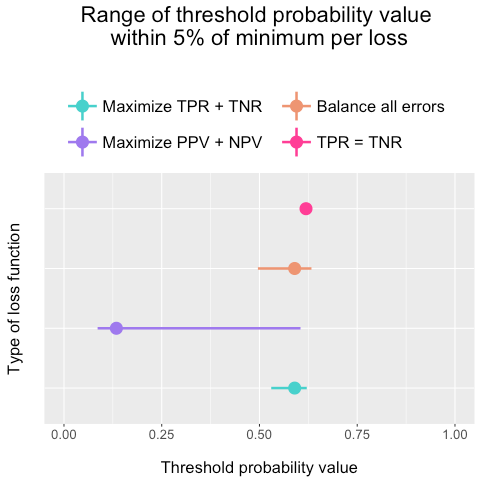## Evaluation data csv

This table (downloadable as csv file) includes all values for TPR, TNR, FPR, FNR, FDR, FOR and the 4 different loss functions (L.diag = maximize TPR+TNR, L.pred = maximize PPV+NPV, L.all = balance all errors, L.eq.diag = equalize FPR and FNR) across the range of threshold probability values (tpv). These are the underlying data of the plots above.